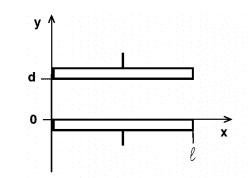# Electric field in a capacitor with a dielectric

## Homework Statement

A parallel plate capacitor has 2 square plates of side l, separated by a distance d (l is a lot bigger than d). Between the plates, there is a linear and non-homogeneous dielectric with constant $$\epsilon_R=1+ay$$ with $$a$$a positive constant. Calculate the electric field between the plates knowing that the capacitor is connected to a battery of voltage V (positive armor at y=0).

Here's an image to help understanding the geometry of the problem:## Homework Equations

Gauss Law:
$$\oint_S {E_n dA = \frac{1}{{\varepsilon _0 }}} Q_{inside}$$

Generalized Gauss law:

kuruman